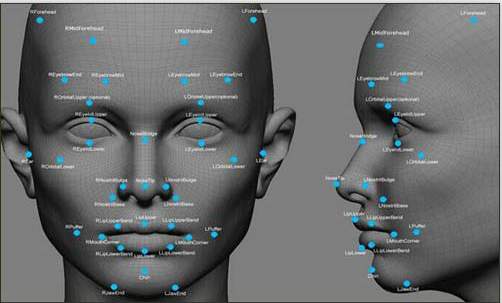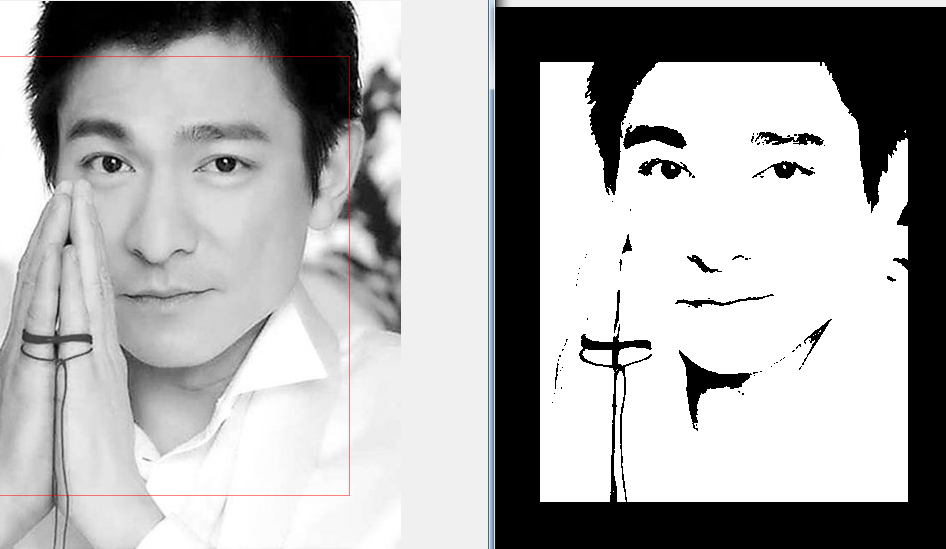﻿ 16074312章安邦人脸识别 - 计算数学达人 - 专，学者，数值代数，微分方程数值解

# 实验三：人脸识别if ~ismatrix(I)
I=rgb2gray(I);%彩色图像灰度化
end;
%I=histeq(I);
%imshow(I);
BW=im2bw(I);%二值化
imshow(BW);%显示二值化后的图片
[n1, n2]=size(BW);%图片长和宽
%把图片分成100块
r=floor(n1/10);%每一小块长度(floor：向下取整)
c=floor(n2/10);%每一小块宽度
x1=1;x2=r;
s=r*c;%每一小块面积
%去边缘
for i=1:10
y1=1;y2=c;
for j=1:10
%if (y2<=c | y2>9*c) | (x1==1 | x2==r*10)
if x1==1||x2==10*r||y1==1||y2==10*c
loc=find(BW(x1:x2, y1:y2)==0);
[o, p]=size(loc);%区域长和宽
pr=o*100/s;
if pr<=100
BW(x1:x2, y1:y2)=0;%把这一小块填充成黑色
r1=x1;r2=x2;s1=y1;s2=y2;%?????
pr1=0;%?????
end
end
y1=y1+c;
y2=y2+c;
end
x1=x1+r;
x2=x2+r;
end
figure,imshow(BW)%显示去边缘后的图片
L=bwlabel(BW,8);%8连通区
BB=regionprops(L, 'BoundingBox');%用来测量标注矩阵L中每一个标注区域的一系列属性
%BoundingBox：包含最小区域的矩形
BB1=struct2cell(BB);%把结构体数组转化为元胞数组
BB2=cell2mat(BB1);%把元胞数组转化为单个矩阵
[s1, s2]=size(BB2);
mx=0;
%??????这个循环是？
for k=3:4:s2-1
p=BB2(1,k)*BB2(1,k+1);
if p>mx && (BB2(1,k)/BB2(1,k+1))<1.8
mx=p;
j=k;
end
end
figure,imshow(I);
hold on;
rectangle('Position',[BB2(1,j-2),BB2(1,j-1),BB2(1,j),BB2(1,j+1)],'EdgeColor','r' )